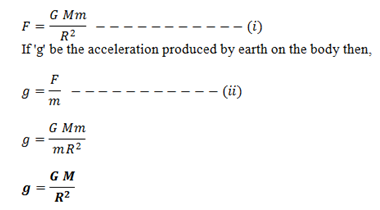# Relation Between ‘g’ & ‘G’

Let us consider a body of mass ‘m’ is placed on the surface of the earth of mass ‘M’ & radius ‘R’. Then from Newton’s law of gravitation,So, from above expression we infer that acceleration due to gravity is dependent only on mass and radius of earth. The ‘g’ doesn’t depend on the mass of body, i.e. in absence of air resistance ‘g’ acts equal for large and small body.